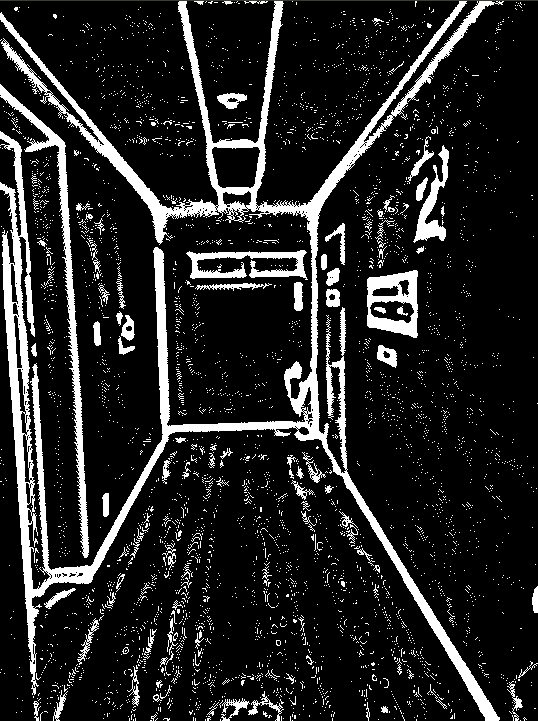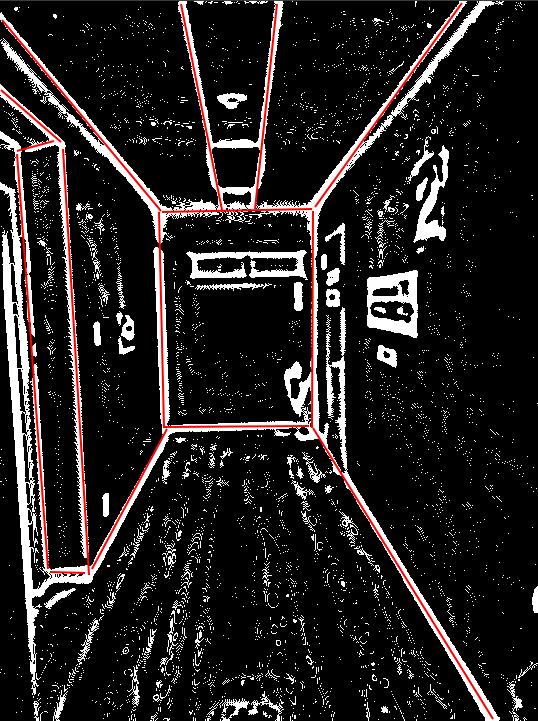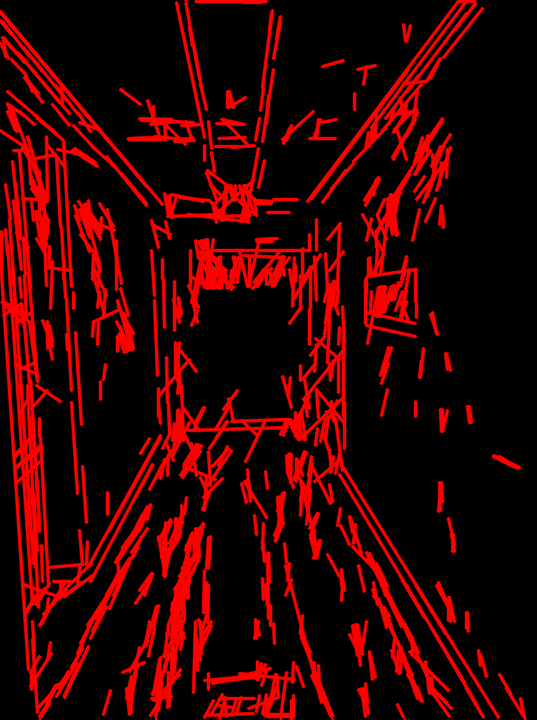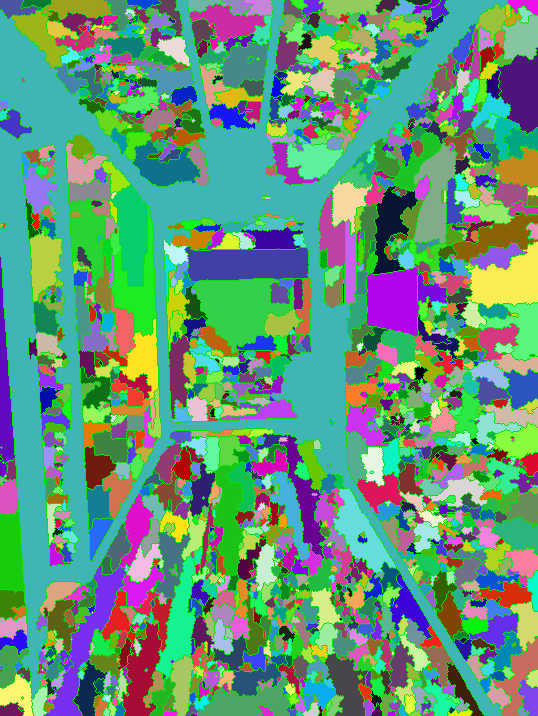# How to draw line?

Hi guys, Have anyone could help me resolve this issue, or have any idea.

After applying a series of algorithms, I have the following result.How to draw line look like bellow.I have to apply HoughLineP but not good.Code  pyrMeanShiftFiltering( img, res, spatialRad, colorRad, maxPyrLevel );

//imshow( "ShiftFiltering", res );

cvtColor(res, res, COLOR_RGBA2GRAY);

GaussianBlur(res, res, Size(15, 15), 0);

//imshow( "GaussianBlur", res );
adaptiveThreshold(res, res, 255, ADAPTIVE_THRESH_GAUSSIAN_C, THRESH_BINARY_INV, 11, 1);
//imshow( "adaptiveThreshold", res );

Mat element = getStructuringElement (MORPH_RECT, Size(3, 3));
morphologyEx(res, res, MORPH_CLOSE, element);

Mat edgs;
Canny(res, edgs, 750, 800, 3);
vector<Vec4f> lines3;
HoughLinesP(edgs, lines3, 1, CV_PI / 180, 20, 15, 10);

Mat markEdges = Mat(res.size(), CV_8UC3);
for (size_t i = 0; i < lines3.size(); i++)
{
Vec4f l = lines3[i];
double dest = euclideanDist(Point2f(l, l), Point2f(l, l));
if (dest > 10) {
line(markEdges, Point2f(l, l), Point2f(l, l), Scalar(0, 0, 255), 2, LINE_AA);
}
}

imshow("markEdges", markEdges);


 I have applied segment image (watershed) but not good.edit retag close merge delete

The HoughLinesP is the way to go. Just check the parameters. If you want to detect ling lines, then set the minLineLength parameter to something bigger (100-150 instead of 15). Use a higher threshold value, too. You can lower the size of the accumulator space to avoid double line detections.

I have config some value, the result is good, but. In my case. I want to detect the edges of the wall after measuring the length of its.

How to merge some line to one line? (look like fitLine method).

Also, before applying the HoughLinesP, like suggested by @kbarni, cleanup your image. There is a lot of noise that can be removed with morphological operations.

1

you can try lsd_lines.cpp instead of HoughLines. it produces better lines to be refined

you can post your own answer if you solved the problem.

Sort by » oldest newest most voted

@sturkmen I have solved this issue following.

The first I have using FastLineDetector/LineSegmentDetector

Above two class has Implementation of merging line segments by TAVARES and PADILHA

Ptr<FastLineDetector> detector;
vector<Vec4i> lines;
detector->detect(grayscale, lines);


Remove small lie if we want.

vector<Vec4i> linesSmall;
copy_if (lines.begin(), lines.end(), back_inserter(linesWithoutSmall), [](Vec4f line){
float length = sqrtf((Point 1, Point 2);
return length > 50;// replace we want to filter.
});


Split your lines using cv::partition. You need to specify a good predicate function. It really depends on the lines which you extract from an image, but I think it should check the following conditions:

• The angle between lines should be quite small (less 3 degrees, for example). Use the dot product to calculate angle's cosine.
• The distance between the centers of segments should be less than half of the maximum length of two segments.

vector<int> labels; int equilavence = partition(linesSmall, labels, {

Extend lines by percentage of line width

float len1 = calculator length of the line using hypot (have in cmath)
float len2 = calculator length of the line using hypot (have in cmath)


Append line if needed using fit line using least-squares problems.

Vec2f lineAfetFit = solve(...)
Vec4i el1 = append line 1;
Vec4i el2 = append line 2;


reject the lines that have wide difference in angles

float a1 = atan(solve(...));
float a2 = atan(solve(...));
if(fabs(a1 - a2) > maxAngleDiff * M_PI / 180.0){
return false;
}


Finds coordinates of perpendicular lines with length d in both line points https://math.stackexchange.com/a/2043...

vector<point2i> boundingLine; after calculating the window around the extended line at least one point needs to inside extended bounding rectangle of other lines.

using  https://docs.opencv.org/3.4.3/d3/dc0/group__imgproc__shape.html#ga1a539e8db2135af2566103705d7a5722
pointPolygonTest(boundingLine, ,...,...);
});


Draw original detected lines

for (int i = 0; i < linesSmall.size(); i++){
Vec4i& line = linesSmall[i];
line(img,
Point(line, line),
Point(line, line), colors[labels[i]], 2);
}


Build point out of each equivalence classes vector<vector<point2i>> pointOut(equilavence);

for (int i = 0; i < linesSmall.size(); i++){
Vec4i& line = linesSmall[i];
pointOut[labels[i]].push_back(Point2i(line, line));
pointOut[labels[i]].push_back(Point2i(line, line));
}


The fit line to each equivalence class point out Using fitLine() pointOut

vector<Vec4i> fittedLines = fitLine(...);


Finished to draw lines after fitting

for(Vec4i line: fittedLines){
line();
}


Resultmore

Official site

GitHub

Wiki

Documentation

## Stats

Asked: 2018-08-29 04:55:26 -0500

Seen: 1,419 times

Last updated: Sep 03 '18